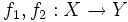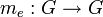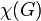# Euler characteristic of compact connected nontrivial Lie group is zero

## Statement

Suppose$G$ is a nontrivial compact connected Lie group (?). Then,$G$ is a Space with zero Euler characteristic (?), i.e., the Euler characteristic (?) of$G$ is$0$.

## Facts used

1. Lefschetz fixed-point theorem
2. Lie group implies polyhedron: Any Lie group can be given a simplicial complex structure, and is hence a polyhedron.
3. Definition of Euler characteristic as the Lefschetz number of the identity map from a space to itself.
4. Lefschetz number is homotopy-invariant: If$f_1,f_2:X \to Y$ are homotopic maps, then the Lefschetz numbers of$f_1$ and$f_2$ are equal.

## Proof

This proof uses a tabular format for presentation. Learn more about tabular proof formats|View all pages on facts with proofs in tabular format

Given: A compact connected nontrivial Lie group$G$.

To prove: The Euler characteristic of$G$ is zero.

Proof: We denote by$e$ the identity element of$G$.

Step no. Assertion/construction Facts used Given data used Previous steps used Explanation
1$G$ is a compact polyhedron, and hence a space with finitely generated homology Fact (2)$G$ is compact and is a Lie group Given+Fact direct
2 Denote by$m_e:G \to G$ the left multiplication map by$e$.$m_e$ is the identity map and the Lefschetz number of$m_e$ is$\chi(G)$ Fact (3)$G$ is a group,$e$ its identity element Step (1) [SHOW MORE]
3 Let$g$ be a non-identity element of$G$. --$G$ is nontrivial
4 Denote by$m_g:G \to G$ the left multiplication map by$g$, a non-identity element of$g$. In other words,$m_g(x) := gx$. Then$m_g$ has no fixed points in$G$ --$G$ is a group Step (3) (basic group theory)
5 The Lefschetz number of$m_g$ is zero. Fact (1) Steps (1), (4) [SHOW MORE]
6$m_e$ and$m_g$ are homotopic maps$G$ is connected and is a manifold (because it's a Lie group) [SHOW MORE]
7 The Lefschetz number of$m_e$ equals the Lefschetz number of$m_g$ Fact (4) -- Step (6) Step+Fact direct
8 The Euler characteristic of$G$ is zero (final conclusion) -- -- Steps (2), (5), (7) [SHOW MORE]